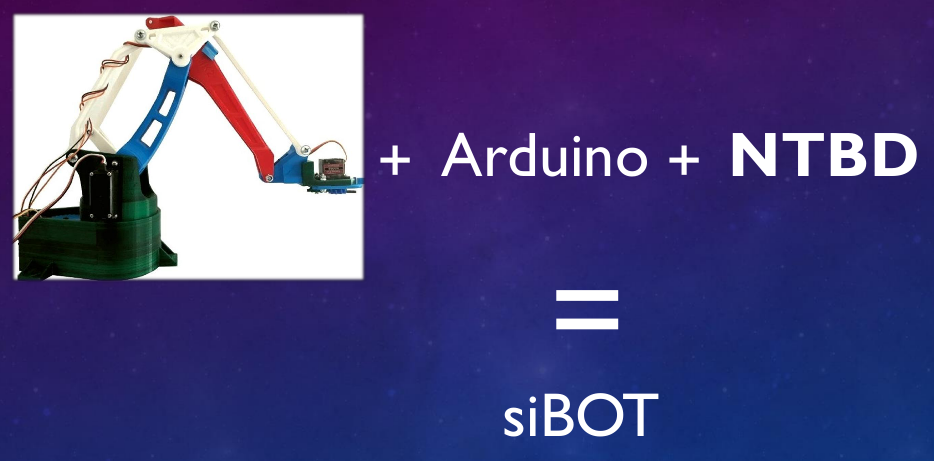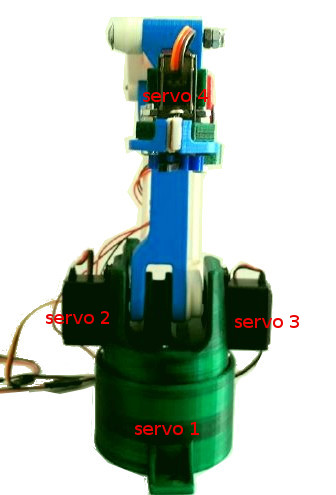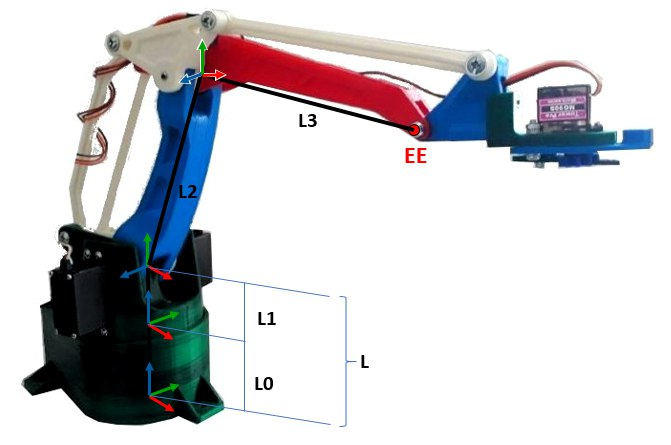# How to control siBOT with the HBR platform

Pubblicato il

In this tutorial we will see how to connect the anthropomorphic manipulator, siBOT, to the HBR platform.

siBOT is the combination of the EEZYBOT MK2 design, italian Open Source project, and of the ROS nodes system needed to control it. I have used this robotic arm for testing the architecture developed during my thesis project, NTBD, about which you can find further info in this post.We will see which steps are needed to connect siBOT in Cloud to control it with HBR platform, either in the joint space (I send desired angles for the motors), either in the task space (in this case I send desired position in the Cartesian space that are then converted into motors’ angles).

# 1. Ingredients

We will need:

• 1 siBOT arm (EEZYBOT MK2 arm + ROS Arduino node)
• 1 Raspberry Pi with HBrain image on its SD

# 2. Control in the motor spaceThe most simple control is that in the motor space: indeed it is sufficient to decide which values we wanto to set for the three servo motors and send them to the robot. We can define a sequence of configurations:

motors_list = [m11,m21,m31,m12,m22,m32,...m1n,m2n,m3n]


and read it correctly in our sketch to send each sequence in a cyclic way in order to make our robot do a sort of “routine”. “.

## 2.1 Desired servo values generator - Sketch

Create a new sketch and call it motors_generator_sibot. The content will have to be the following:

import dotbot_ros
from std_msgs.msg import Int16MultiArray
import time

class Node(dotbot_ros.DotbotNode):
node_name = 'motors_generator'

def setup(self):
self.pub = dotbot_ros.Publisher('motors_nointerp', Int16MultiArray)
self.gripper = 25

motors_list = [90, 90, 90, 180, 100, 30, 0, 140, 60]
self.gripp_list = ['open','closed','open']
n = 3
self.m_list = [motors_list[i:i+n] for i in range(0, len(motors_list), n)]
self.loop_rate = dotbot_ros.Rate(0.33)

def loop(self):
for indx, motors_seq in enumerate(self.m_list):
seq = Int16MultiArray()
if self.gripp_list[indx] == 'open':
self.gripper = 100
else:
self.gripper = 25
seq.data = [m for m in motors_seq, self.gripper]
self.pub.publish(seq)
time.sleep(3)


## 2.2 Desired servo values generator - Sketch with comments

Here I provide the code with comments. As you can see, the structure is that used since now for the ROS nodes on HBrain: functions setup() and loop() are defined with the addition of a callback function.

import dotbot_ros
from std_msgs.msg import Int16MultiArray
import time

class Node(dotbot_ros.DotbotNode):
node_name = 'motors_generator'

def setup(self):
# We define a publisher to publish the motors sequences
self.pub = dotbot_ros.Publisher('motors_nointerp', Int16MultiArray)
# Initialize the value for the gripper as closed.
self.gripper = 25
# Sequence of desired angles (to be read 3 at a time)
motors_list = [90, 90, 90, 180, 100, 30, 0, 140, 60]
# Sequence of desired gripper configurations
self.gripp_list = ['open','closed','open']
# As said, we take the values in groups of n elements
n = 3
# The following code returns a list of lists each one of 3 elements:
# [[motor_seq1], [motor_seq2],...[motor_seqn]]
self.m_list = [motors_list[i:i+n] for i in range(0, len(motors_list), n)]
self.loop_rate = dotbot_ros.Rate(0.33)

def loop(self):
# During the for cycle in the list of lists, I save also the index of each
# element so as to cycle in my list of gripper values.
for indx, motors_seq in enumerate(self.m_list):
seq = Int16MultiArray()
if self.gripp_list[indx] == 'open':
self.gripper = 100
else:
self.gripper = 25
seq.data = [m for m in motors_seq, self.gripper]
# We publish on the topic defined by pub the sequence of motors,
# combination of the joints mini-servo angles and the gripper value.
self.pub.publish(seq)
time.sleep(3)


# 3. Control of the End Effector position

We have seen how to control siBOT’s motors values, but often in industrial robotics the aim is to control the position and orientation of the End Effector (EE) that in this case corresponds to the gripper joint.In order to obtain the desired position it is necessary to manipulate the latter information and transform it into values for the robotic arm motors, which are the only way to move the robot itself. This operation is called inverse kinematics and, through some computations, analytical or numerical, allows to find the values of the motors corresponding to a certain EE position. Note that in this case we can only define the desired position (not the orientation) since the gripper does not rotate sdue to the arm physical configuration. Also in this case we define a sequence of desired positions that we’d like the robot EE to reach.

## 3.1 End Effector positions generator - Sketch

Create a new sketch and call it positions_generator_sibot. The content will have to be the following:

import dotbot_ros
from geometry_msgs.msg import Point
from std_msgs.msg import String
import time

class Node(dotbot_ros.DotbotNode):
node_name = 'position_generator'

def setup(self):
self.pub = dotbot_ros.Publisher('desired_position_nointerp', Point)
self.pubG = dotbot_ros.Publisher('gripper_value', String)

desPos = [150, 0, 235, 130, 50, 140, 130, -30, 90]
self.gripp_list = ['open','closed','open']
n = 3
self.desP = [desPos[i:i+n] for i in range(0, len(desPos), n)]
self.loop_rate = dotbot_ros.Rate(0.33)

def loop(self):
for indx,pos in enumerate(self.desP):
self.pubG.publish(self.gripp_list[indx])
des_pos =  Point(*pos)
self.pub.publish(des_pos)
time.sleep(3)


## 3.2 End Effector positions generator - Sketch with comments

As you can notice, in the case of desired positions, we don’t have the callback function called as new messages are received on the topic inwhich the gripper status is published (gripper_value): this function will be defined in the Inverse Kinematics node within which the servo-motors values together with the gripper value are published on the specific topic.

import dotbot_ros
from geometry_msgs.msg import Point
import time

class Node(dotbot_ros.DotbotNode):
node_name = 'position_generator'

def setup(self):
# We define a publisher to publish the EE positions sequence and
# one for the gripper value
self.pub = dotbot_ros.Publisher('desired_position_nointerp', Point)
self.pubG = dotbot_ros.Publisher('gripper_value', String)
# Positions in Cartesian coordinates expressed in millimeters.
# (to be read as [x1,y1,z1,x2,y2,z2...xn,yn,zn])
desPos = [150, 0, 235, 130, 50, 140, 130, -30, 90]
# Sequence of desired configurations for the gripper
self.gripp_list = ['open','closed','open']
# As said, we take the values in groups of n elements
n = 3
# The following code returns a list of lists of 3 elements.
# Each list is a position in Cartesian coordinates:
# [[pos1], [pos2],...[posn]]
self.desP = [desPos[i:i+n] for i in range(0, len(desPos), n)]
self.loop_rate = dotbot_ros.Rate(0.33)

def loop(self):
# During the for cycle in the list of lists, i savce also the index
# of each element so as to cycle in the gripper values list
for indx,pos in enumerate(self.desP):
# We publish the string to define if the gripper must be open or closed
# in that configuration, on the topic gripper_value
self.pubG.publish(self.gripp_list[indx])
des_pos =  Point(*pos)
# We publish on the topic defined by pub, the desired position, as Point message
self.pub.publish(des_pos)
time.sleep(5)


## 3.3 Inverse kinematics node - Sketch

Once the desired positions will be published, it will be necessary to convert it in the correspondent servo values. Many solutions exist, depending on the complexity of the problem ( e.g., the Degrees Of Freedom) but my solution is a geometrical one, still feasible having the arm only 3 DOF. Create a new sketch and call it IK_sibot. The content will have to be the following:

import dotbot_ros
import math
from std_msgs.msg import Int16MultiArray
from std_msgs.msg import String
from geometry_msgs.msg import Point
from sys import stdout

class Node(dotbot_ros.DotbotNode):
node_name = 'IK'

def setup(self):
dotbot_ros.Subscriber("desired_position", Point, self.callback)
dotbot_ros.Subscriber("gripper_value", String, self.gripper_callback)
self.pub = dotbot_ros.Publisher('motors', Int16MultiArray)
self.gripper = 25

def hipo(self,x,y):
return math.sqrt(x*x + y*y)

def lawOfCosines(self,a,b,c):
rate = (a*a + b*b - c*c) / (2 * a * b)
if abs(rate) > 1:
if max(rate,0) == 0:
rate = -1
if max(rate,0) == rate:
rate = 1
return math.acos(rate)

return rad * 180 / math.pi

def gripper_callback(self, msg):
if msg.data == "open":
self.gripper = 100
else:
self.gripper = 25

def callback(self,data):
L0 = 50
L1 = 35
L2 = 150
L3 = 150
cartP = {'xEE':data.x, 'yEE': data.y, 'zEE': data.z}

L = L0 + L1
cylP = {'theta': math.atan(cartP['yEE']/cartP['xEE']), 'r':self.hipo(cartP['xEE'], cartP['yEE']), 'zhat':cartP['zEE']-L}
zhat = cylP['zhat']
rho = self.hipo(cylP['r'], zhat)

M1 = 2*cylP['theta'] + math.pi/2
M2 = math.atan(zhat/cylP['r']) + self.lawOfCosines(L2,rho,L3)
M3 = M2 + self.lawOfCosines(L2,L3,rho) - math.pi/2
angles = [M1,math.pi - M2,M3]
values = Int16MultiArray()
values.data = [self.deg(angle) for angle in angles]
values.data.append(self.gripper)

if values.data > 180:
values.data = 180
print " motor 1 has been saturated!"
if values.data > 145:
values.data = 145
print " motor 2 has been saturated!"
if values.data < 55:
values.data = 55
print " motor 2 has been saturated!"
if values.data > 110:
values.data = 110
print " motor 3 has been saturated!"
if values.data < 20:
values.data = 20
print " motor 3 has been saturated!"
stdout.flush()
self.pub.publish(values)



## 3.4 Inverse kinematics node - Sketch with comments

import dotbot_ros
import math
from std_msgs.msg import Int16MultiArray
from std_msgs.msg import String
from geometry_msgs.msg import Point
from sys import stdout

class Node(dotbot_ros.DotbotNode):
node_name = 'IK'

def setup(self):
dotbot_ros.Subscriber("desired_position", Point, self.callback)
dotbot_ros.Subscriber("gripper_value", String, self.gripper_callback)
self.pub = dotbot_ros.Publisher('motors', Int16MultiArray)
self.gripper = 25

# Here are defined some useful functions for the Inverse Kinematics problem solution
def hipo(self,x,y):
return math.sqrt(x*x + y*y)

def lawOfCosines(self,a,b,c):
rate = (a*a + b*b - c*c) / (2 * a * b)
if abs(rate) > 1:
if max(rate,0) == 0:
rate = -1
if max(rate,0) == rate:
rate = 1
return math.acos(rate)

return rad * 180 / math.pi
# Callback function to set the servo motors of the gripper,
# depending on the published string in gripper_value
def gripper_callback(self, msg):
if msg.data == "open":
self.gripper = 100
else:
self.gripper = 25
# Callback function, called when a desired position is published on
# the specific topic, in which the values for the servos are computed
# and combined with the gripper value.
def callback(self,data):
L0 = 50
L1 = 35
L2 = 150
L3 = 150
# Desired position derived from the Point type message
cartP = {'xEE':data.x, 'yEE': data.y, 'zEE': data.z}
L = L0 + L1
# Desired position in cylindrical coordinates
cylP = {'theta': math.atan(cartP['yEE']/cartP['xEE']), 'r':self.hipo(cartP['xEE'], cartP['yEE']), 'zhat':cartP['zEE']-L}
zhat = cylP['zhat']
rho = self.hipo(cylP['r'], zhat)

M1 = 2*cylP['theta'] + math.pi/2
M2 = math.atan(zhat/cylP['r']) + self.lawOfCosines(L2,rho,L3)
M3 = M2 + self.lawOfCosines(L2,L3,rho) - math.pi/2

angles = [M1,math.pi - M2,M3]
values = Int16MultiArray()
values.data = [self.deg(angle) for angle in angles]
values.data.append(self.gripper)
# We limit the motors valuesto the limits defined by the physical structure of the robot
if values.data > 180:
values.data = 180
print " motor 1 has been saturated!"
if values.data > 145:
values.data = 145
print " motor 2 has been saturated!"
if values.data < 55:
values.data = 55
print " motor 2 has been saturated!"
if values.data > 110:
values.data = 110
print " motor 3 has been saturated!"
if values.data < 20:
values.data = 20
print " motor 3 has been saturated!"
stdout.flush()
# We publish, on the topic defined by pub, the sequence of motors, combination of the joint miniservo angles and the gripper value.
self.pub.publish(values)


# 4. Interpolation

The nodes for controlling the manipulator are ready but a node is missing, with the role of making the movements from one configuration to the other smoother: an interpolation node. For sake of simplicity we assume that no obstacles are present and we implement a linear interpolation. we thus implement a very simple path planning node.

## 4.1 Path Planning node - Sketch with comments

I will directly provide the commented code. Copy and paste this code in a sketch called linear_interp_sibot.

import dotbot_ros
import math
from geometry_msgs.msg import Point
from std_msgs.msg import Int16MultiArray
import time

class Node(dotbot_ros.DotbotNode):
node_name = 'interpolator'

def setup(self):
# We define the publisher and the subscribers
self.pub = dotbot_ros.Publisher('desired_position', Point)
self.pubM = dotbot_ros.Publisher('motors', Int16MultiArray)
dotbot_ros.Subscriber("desired_position_nointerp", Point, self.callback)
dotbot_ros.Subscriber("motors_nointerp", Int16MultiArray, self.motors_callback)
self.i = 0
self.pointA = Point()
self.pointB = Point()
self.motorA = Int16MultiArray()
self.motorB = Int16MultiArray()
# Here some useful functions for the computation of the interpolation points are defined
def coord_distance_AB(self,a,b):
d = Point()
d.x = abs(b.x-a.x)
d.y = abs(b.y-a.y)
d.z = abs(b.z-a.z)
return d

def values_distance_AB(self,a,b):
d = Int16MultiArray()
d.data.append(abs(b.data-a.data))
d.data.append(abs(b.data-a.data))
d.data.append(abs(b.data-a.data))
return d

# Callback function called when the motors sequence is published
# It reads the current sequence then compares it with the previous one to compute the intermediate values in 20 steps (N=20)
def motors_callback(self,data):
N = 20
self.i += 1
if self.i == 1:
self.motorA = data
self.pubM.publish(self.motorA)
else:
self.motorB = data
d = self.values_distance_AB(self.motorA, self.motorB)
if d.data == 0 and d.data == 0 and d.data == 0 and d.data == 0 :
self.motorA = self.motorB
self.pubM.publish(self.motorA)
else:
for self.i in range(1,N+1):
M = Int16MultiArray()
if self.motorA.data < self.motorB.data:
M.data.append(self.motorA.data + d.data/N)
else:
M.data.append(self.motorA.data - d.data/N)
if self.motorA.data < self.motorB.data:
M.data.append(self.motorA.data + d.data/N)
else:
M.data.append(self.motorA.data - d.data/N)
if self.motorA.data < self.motorB.data:
M.data.append(self.motorA.data + d.data/N)
else:
M.data.append(self.motorA.data - d.data/N)

M.data.append(self.motorB.data)

self.pubM.publish(M)
self.motorA = M
self.i += 1
time.sleep(0.05)
# Callback function called when a new EE position is published
# It reads the current position then compares it to the previous one in order to
# compute the intermediate values in 20 steps (N=20)
def callback(self,data):
N = 20
self.i += 1
if self.i == 1:
self.pointA = data
self.pub.publish(self.pointA)
else:
self.pointB = data
d = self.coord_distance_AB(self.pointA, self.pointB)
if d.x == 0 and d.y == 0 and d.z == 0:
self.pointA = self.pointB
self.pub.publish(self.pointA)
else:
for self.i in range(1,N+1):

P = Point()

if self.pointA.x < self.pointB.x:
P.x = self.pointA.x + d.x/N
else:
P.x = self.pointA.x - d.x/N

if self.pointA.y < self.pointB.y:
P.y = self.pointA.y + d.y/N
else:
P.y = self.pointA.y - d.y/N

if self.pointA.z < self.pointB.z:
P.z = self.pointA.z + d.z/N
else:
P.z = self.pointA.z - d.z/N
self.pub.publish(P)
self.pointA = P
self.i += 1
time.sleep(0.05)


RMK: ithe values for the servos can only be integers, the interpolation thus generates values which may be different from the desired ones.

# 5. Arduino Sketch

In order to control the manipulator it is necessary to control its motors with an Arduino board on which a serial ROS node will be run which will read the messages on the topics we want it to read on. Load the following sketch on your Arduino board:

/*
* siBOT servo control
*/
#include <Servo.h>
#include <ros.h>
#include <std_msgs/Int16MultiArray.h>
#define USE_USBCON

ros::NodeHandle  nh;

Servo servo1, servo2, servo3, servo4;

// Callback function (when a new sequence of motors is published
// write the angles on the respective pins)
void motors_cb( const std_msgs::Int16MultiArray& angles_msg){

servo1.write(angles_msg.data);
servo2.write(angles_msg.data);
servo3.write(angles_msg.data);
servo4.write(angles_msg.data);

}

// Subscriber definition. Note that the topic is specific to the robot on which the Ros nodes are running (/hotbot/ in this case)
ros::Subscriber<std_msgs::Int16MultiArray> sub("/hotbot/motors",motors_cb);

void setup(){

nh.initNode();
nh.subscribe(sub);

servo1.attach(2); //attach it to pin 2
servo2.attach(3);
servo3.attach(4);
servo4.attach(5);
}

void loop(){
nh.spinOnce();
delay(1);
}


# 6. Rosserial start and nodes run

So as to advertise on the ROS system the serial node running on the Arduino, a specific python serial node must be launched. To do so through the paltform, it is necessary to open this link and click on “Apri Manager Robot”. Once the page is open, click on start where “rosserial” appears in order to launch the serial node.

As the serial node is launched, we just have to run the necessary nodes fot he robot control, depending on the chosen control:

• Control in the joint space: motors_generator_sibot, linear_interp_sibot
• Control of the EE positionControllo della posizione dell’EE: positions_generator_sibot, IK_sibot, linear_interp_sibot

# 7. Exercises

• Write a sketch called set_motors_sibot which sends a sequence of motors for each loop() execution.
• Write a sketch called set_position_sibot which send an EE position at each loop() execution.

Hint: exploit the porvided code and modify it to make it more simpler and use it as a base to publish on the right topics. Furthermore, to make the interpolation be executed, it is sfficient to run the linear_interp_sibot sketch in addition to the sketch you will write.

Bye bye!